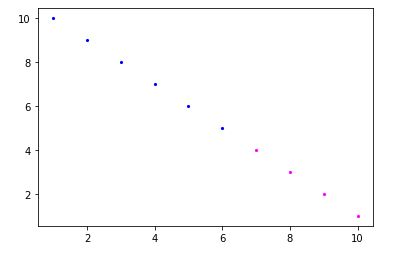# Mark different color points on matplotlib

• Last Updated : 03 Jun, 2020

Matplotlib is an amazing visualization library in Python for 2D plots of arrays. Matplotlib is a multi-platform data visualization library built on NumPy arrays and designed to work with the broader SciPy stack.

In this article, the task is to mark different color points in a graph based on a condition that the values of the elements of the list say x is less than or equal to 7 should be colored in blue and those greater should be colored magenta. The idea is to plot a graph having points grouped under one condition in different colors, basically to group the clusters in one color. For this, we run a loop for all values of x and assign a color value to the corresponding value of x. A list will be made of blue and magenta colors specifying the color at the ith index.

Below is the implementation.

 `import`  `numpy as np``import` `matplotlib.pyplot as plt`` ` ` ` `# values of x``x ``=` `np.array([``1``, ``2``, ``3``, ``4``, ``5``,``              ``6``, ``7``, ``8``, ``9``, ``10``])`` ` `# values of y``y ``=` `np.array([``10``, ``9``, ``8``, ``7``, ``6``, ``5``,``              ``4``, ``3``, ``2``, ``1``])`` ` `# empty list, will hold color value``# corresponding to x``col ``=``[]`` ` `for` `i ``in` `range``(``0``, ``len``(x)):``    ``if` `x[i]<``7``:``        ``col.append(``'blue'``)  ``    ``else``:``        ``col.append(``'magenta'``) `` ` `for` `i ``in` `range``(``len``(x)):``     ` `    ``# plotting the corresponding x with y ``    ``# and respective color``    ``plt.scatter(x[i], y[i], c ``=` `col[i], s ``=` `10``,``                ``linewidth ``=` `0``)``     ` ` ` `plt.show()`

Output:My Personal Notes arrow_drop_up